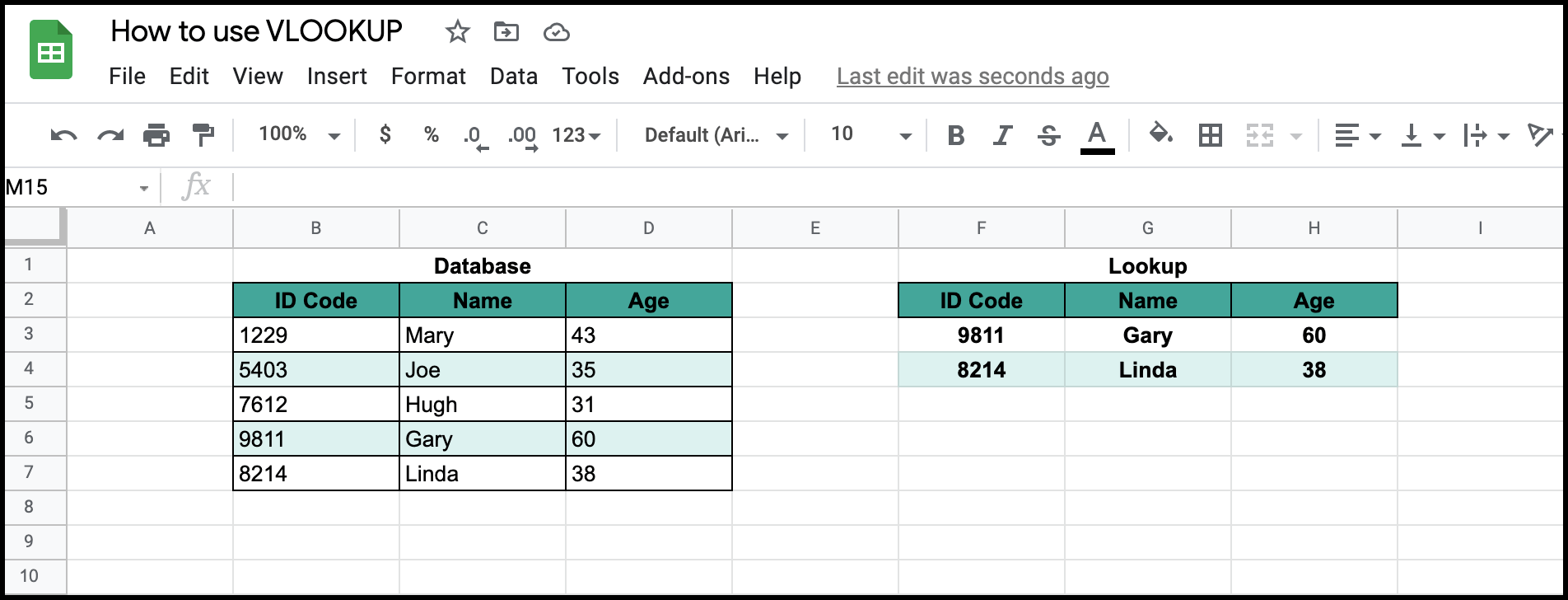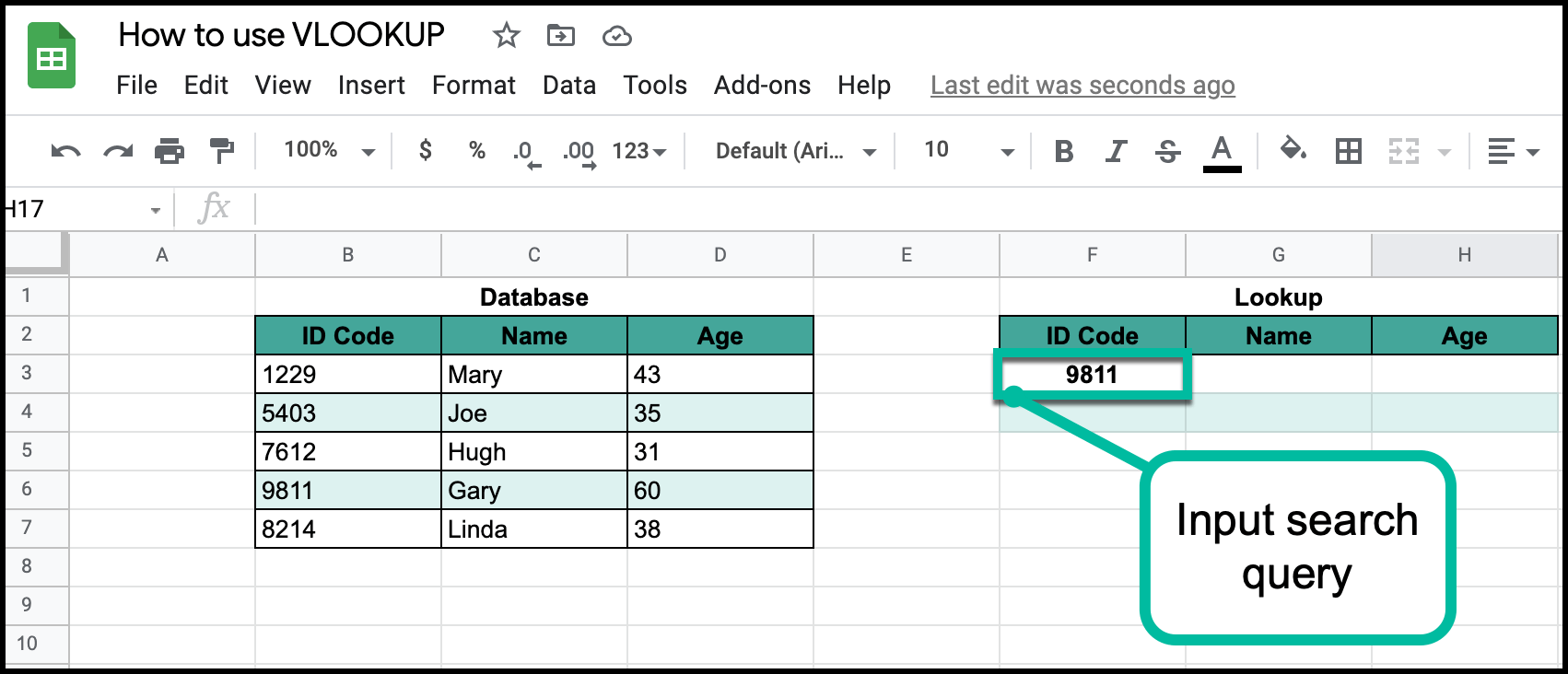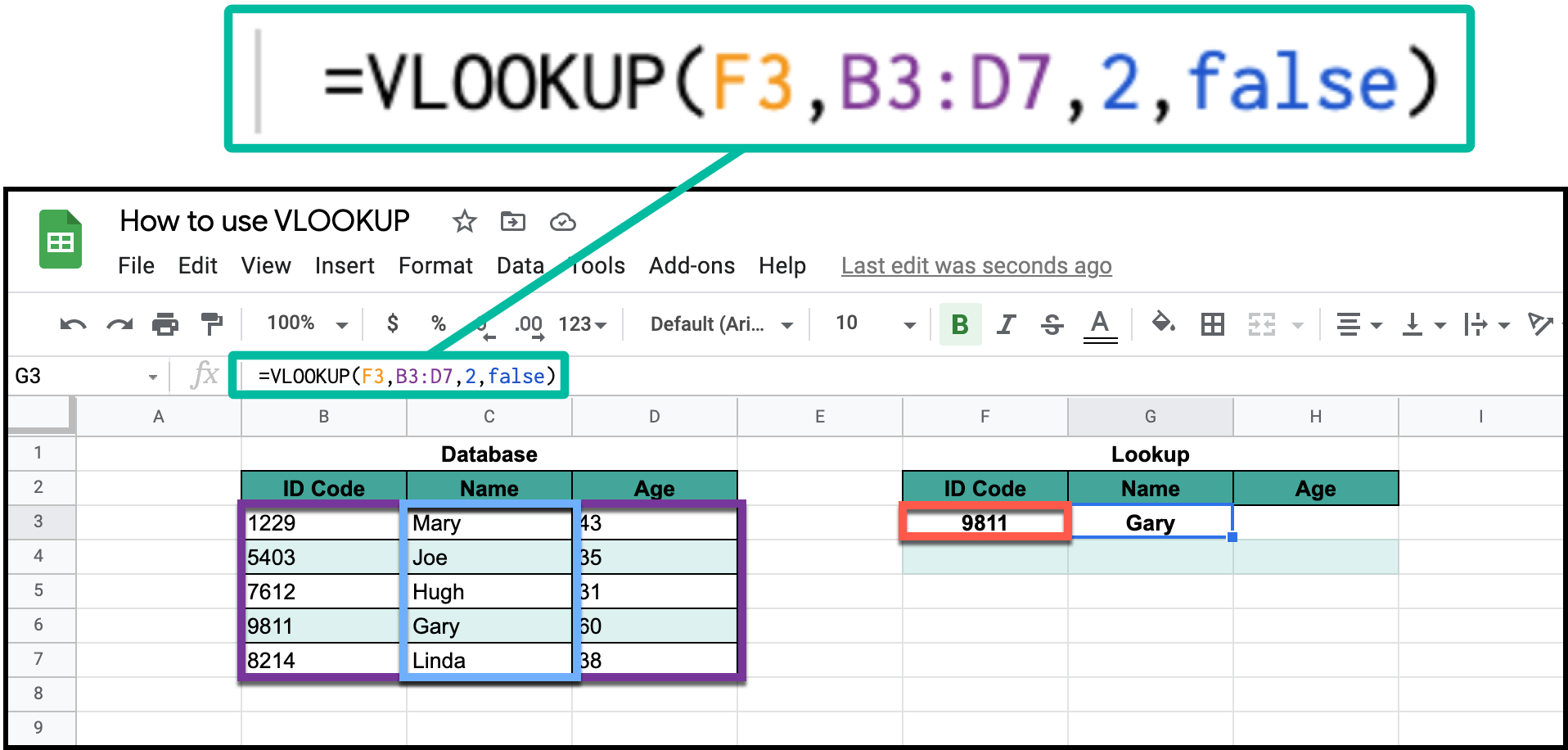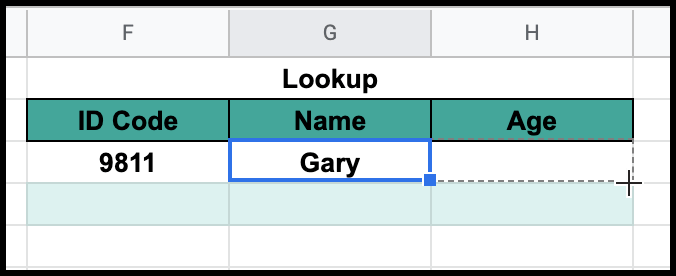# How to Use the VLOOKUP Function in Google SheetsIn Google Sheets, lookup functions are super useful. The "VLOOKUP", or Vertical Lookup, is a google sheets lookup function that lets you pull information from massive ranges of data for quick and rapid analysis. Today, I will show you how to both use and understand this feature.

If you have a horizontal data table, you will want to use HLOOKUP (Horizontal Lookup).

## Step #1: Input Search QueryFirst, let's assume that the data table on the left side of the screen is the database and the data table on the right is where you look up the info from said database. Start out by inputing the leftmost column value of a random person in the group, let's say 9811 (Gary).

## Step #2: "=VLOOKUP()"The vlookup function is definitely one of the more confusing ones out there, but it makes more sense once you break it down into its separate parts.

1. Type in "=VLOOKUP(".
2. Reference the "key" cell that will be searched upon (in this case, cell F3) followed by a comma.
3. Reference the data table's range (in this case, B3 to D7) followed by a comma.
4. Select what column you want to have displayed (1 is ID Code, 2 is Name, 3 is Age). followed by a comma.
5. Type the word "false". This will set up a conditional statement that insures that the only value that will be displayed is the one that truly matches the parameters of the search.
6. End the function with a closing parentheses and hit ENTER to lookup the value.

If you enter the formula wrong, you will get a formula parse error, meaning that there was something wrong with your function.

## Step #3: Drag and FillNow, to fill in the rest of the data, all you have to do is to click and drag the equation cell to the right and it will come back to you with Gary's age, 60.

And there you have it; that is how you use VLOOKUP in Google Sheets.